# GSEB Solutions Class 11 Chemistry Chapter 7 Equilibrium

Gujarat Board GSEB Textbook Solutions Class 11 Chemistry Chapter 7 Equilibrium Textbook Questions and Answers.

## Gujarat Board Textbook Solutions Class 11 Chemistry Chapter 7 Equilibrium

### GSEB Class 11 Chemistry Equilibrium Text Book Questions and AnswersQuestion 1.

A liquid is in equilibrium with its vapour in a sealed container at a fixed temperature. The volume of the container is suddenly increased.
(a) What is the initial effect of the change on vapour pressure?
(b) How do rates of evaporation and condensation change initially?
(c) What happens when equilibrium is restored finally and what will be the final vapour pressure?
(a) The vapour pressure on the surface of the liquid initially decreases by the sudden increase in the volume of the container. V.P. will decrease.

(b) On increasing the volume of the container suddenly, initially the rate of evaporation remains constant. However, due to increase in the volume of the vapour, the rate of condensation decreases or it will be low.

(c) When equilibrium is restored finally, the rate of evaporation will again be equal to the rate of condensation. The final vapour pressure will be the same as it was before the sudden increase in the volume of the container as V.P. does not depend upon volume.Question 2.
What is KC for the following equilibrium when the equilibrium concentration of each substance is : [SO2] = 0.60 M, [OC] = 0.82 M and [SO3] = 1.90 M?
2SO2(g) +O2(g) ⇌ 2SO3(g)
Solution: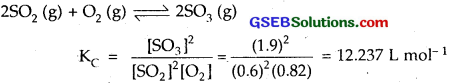Question 3.
At a certain temperature and total pressure of 105 Pa, iodine vapour contains 40% by volume of I atoms
I2(g) ⇌2I (g)
Calculate Kp for the equilibrium.
Solution:
I2(g) ⇌ 2I (g)
Total pressure = 105 Pa
Since out of total volume, 40% by volume are I atoms
∴ 60% are I2 molecules (gaseous)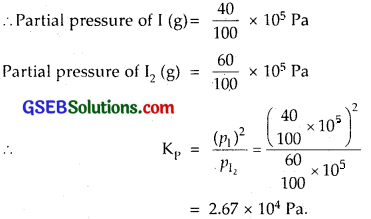Question 4.
Write the expression for the equilibrium constant, KC for each of the following reactions :
(i) 2NOCl(g) ⇌ 2NO (g) + Cl2 (g)
(ii) 2Cu[NO3]2 (s) ⇌ 2CuO (s) + 4NO2 (g) + O2 (g)
(iii) CH3COOC2H5 (aq) + H2O (l) ⇌ CH3COOH (aq) + C2H5OH (aq)
(iv) Fe3+ (aq) + 3OH (aq) Fe(OH)3 (s)
(v) I2 (s) + 5F2 ⇌ 2IF5.
Solution:
(i) KC = $$\frac{[\mathrm{NO}]^{2}\left[\mathrm{Cl}_{2}\right]}{[\mathrm{NOCl}]^{2}}$$ where, [NO], [NOCl] represents molar concentfations.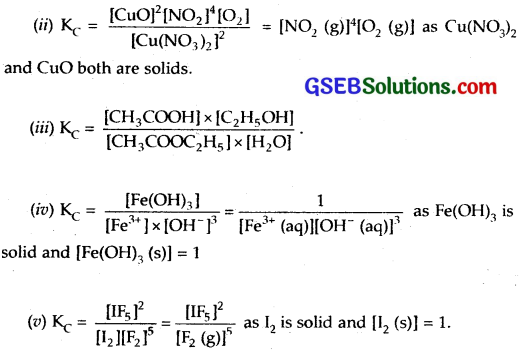Question 5.
Find out the value of KC for each of the following equilibria from the value of K .
(i) 2NOCl (g) ⇌ 2NO (g) + Cl2(g); Kp = 1.8 x 10-2 at 500 K
(ii) CaCO3 (s) ⇌ CaO (s) = CO2 (g); Kp = 167 at 1073 K
Solution:
(i) 2NOCl (g) ⇌ 2NO (g) + Cl2 (g)
Kp = 1.8 x 10-2 at 500 K
∆n = change in the no. of moles (gaseous)
= [2 + 1] – 2 = 1
∴ K = KC x (RT)∆n [ R = 0.0821 L atm mol-1 ]
or 1.8 x 10-2 = KC x (RT)1
or KC = $$\frac{1.8 \times 10^{-2}}{\mathrm{RT}}$$
= $$\frac{1.8 \times 10^{-2}}{0.0821 \times 500}$$
= 4.4 x 10-4

(ii) CaCO3(s) ⇌ CaO (s) + CO2(g)
Kp = 167 at 1073 K; Here ∆n = 1
∴KC = $$\frac{K_{p}}{R T}$$
= $$\frac{167}{0.0821 \times 1073}$$
= 1.90Question 6.
For the following equilibrium, KC = 6.3 x 1014 at 1000 K
NO (g) + O3(g) ⇌ NO2(g) + O2(g)
Both the forward and reverse reactions in the equilibrium and elementary bimolecular reactions. What is KC, for the reverse reaction?
Solution:
KC = 6.3 x 1014 at 1000 K for the equilibrium
NO (g) + O3 (g) ⇌ NO2 (g) + O2 (g)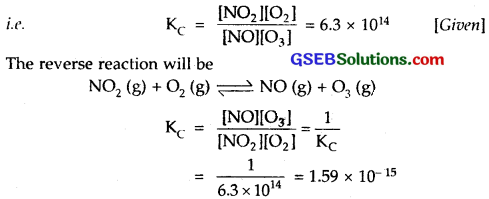Question 7.
Explain why pure liquids and solids can be ignored while writing the equilibrium constant expression?
By convention active mass of a pure solid is constant irrespective of its amount and if a pure liquid is present in excess (e.g. as a solvent) its active mass is also constant. In either case, we put their active mass equal to 1 (because their constant values are included in the equilibrium constant)
[Pure solid] = 1, [Pure liquid] = 1.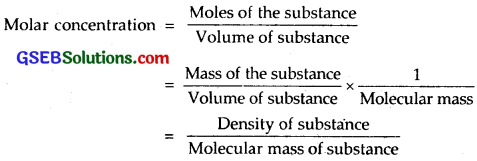As density of a particular pure substance at a particular temperature is constant (being an intensive property depending only on the nature of the substance and not on the amount) and molecular mass of a substance is also constant, therefore, molar concentration is constant.

Question 8.
Reaction between N2 and O2 takes place as follows:
2N2 (g) + O2 (g) ⇌ 2N2O (g)
If a mixture of 0.482 mol N2 and 0.933 mol of O2 is placed in a 10 L reaction vessel and allowed to form N2O at a temperature for which KC = 2.0 x 10-37, determine the composition of equilibrium mixture.
Solution:
Given reaction is
2N2 (g) + O2 (g) ⇌ 2N2O(g)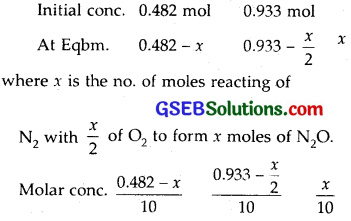As KC = 2.0 x 10-37 is very small, this means that the amount of N2 and O2 reacted (x) is very small.
Hence at equilibrium, we have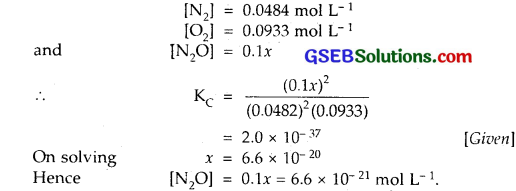Question 9.
Nitric oxide reacts with Br2 and gives nitrosyl bromide as per reaction given below :
2NO (g) + Br2 (g) ⇌ 2NOBr (g)
When 0.087 mol of NO and 0.0437 mol of Br2 are mixed in a closed container at constant temperature, 0.0518 mol of NOBr is obtained at equilibrium. Calculate equilibrium amount of NO and Br2.
Solution:
2NO (g) + Br2 (g) ⇌ 2NOBr (g)
Initial cone. 0.087 mol 0.0437 mol
Final cone. ? ? 0.0518
Equilibrium cone. (0.087 – 0.0518) (0.0437 – 0.0259)
= 0.0352 mol = 0.0178 mol
∴ Equilibrium amount of [NO] = 0.0352 mol
Equilibrium amount of [Br2] = 0.0178 mol.Question 10.
At 450 K, Kp = 2.0 x 1010/bar for the given reaction at equilibrium
2SO2 (g) + O2 (g) ⇌ 2SO3 (g)
What is KC at this temperature?
Solution:
The given equation is
2SO2 (g) + Oz (g) ⇌ 2SO3 (g)
∆n = 2 – [2 + 1]= – 1
∴ Kp = KC x (RT)-1
∴KC = Kp x (RT)-1
= 2.0 x 1010 x 0.0831 x 450
= 7.47 x 1011L mol-1
∴ KC for the reaction at 450 K = 7.47 x 1011 L mol-1.

Question 11.
A sample of HI (g) is placed in flask ac a pressure of 0.2 atm. At equilibrium the partial pressure of HI (g) is 0.04 atm. What is Kp for the given equilibrium?
2HI (g) H2 (g) + I2 (g)
Solution:
2HI (g) H2 (g) ⇌ H2 (g) + I2
At eqbm. pm = 0.04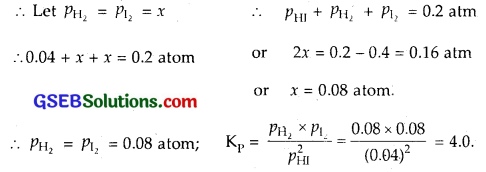Question 12.
A mixture of 1.57 mol of N2, 1.92 mol of H2 and 8.13 mol of NH3 is introduced into a 20 L reaction vessel at 500 K. At this temperature, the equilibrium constant, KC for the reaction N2 (g) + 3H2 (g) ⇌ 2NH3 (g) is 1.7 x 1O2. Is the reaction mixture at equilibrium? If not, what is the direction of the net reaction?
Solution:
The given equation for the reaction between N2 and H2 to form NH3 is
N2 (g) + 3H2 (g) ⇌ 2NH3(g)
KC for the reaction is 1.7 x 102
Concentration quotient (QC) for the reaction at any stage of the reaction, other than the chemical equilibrium is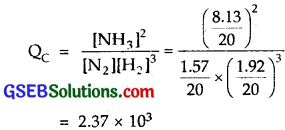= 2.37 x 103
Since QC = 2.37 x 103 and KC = 1.7 x 102
QC # KC. The reaction mixture is not at equilibrium. As QC > KC.
∴ QC will tend to decrease so as to become equal to K.
As a result the direction of the net reaction will be backwards.Question 13.
The equilibrium constant expression for a gas reaction is,
K = $$\frac{\left[\mathrm{NH}_{3}\right]^{4}\left[\mathrm{O}_{2}\right]^{5}}{[\mathrm{NC}]^{4}\left[\mathrm{H}_{2} \mathrm{O}\right]^{6}}$$
Write the balanced chemical equation corresponding to this expression.
Solution:
Here NH3 and O2 are products and NO and H2O are reactants.
∴ The balanced chemical equation will be
4NO + 6H2 ⇌ 4NH3 + 6O2

Question 14.
One mole of H2O and one mole of CO are taken in 10 L vessel and heated to 725 K. At equilibrium 40% of water (by mass) reacts with CO according to the equation.
H2O (g) + CO (g) ⇌ H2 (g) + CO2 (g)
Calculate the equilibrium constant for the reaction.
Solution: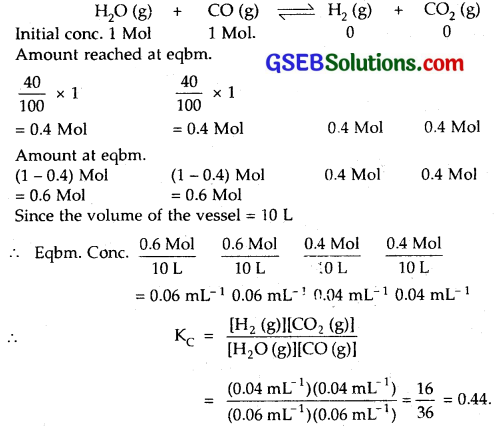Question 15.
At 700 K, equilibrium constant for the reaction :
H2 (g) + I2 (g) ⇌ 2HI (g)
is 54.8. If 0.5 mol L-1 of HI (g) is present at equilibrium at 700 K, what are the concentration of H2 (g) and I2 (g) assuming that we initially started with HI (g) and allowed it to reach equilibrium at 700 K?
Solution:
The equilibrium constant (KC) for the reaction
H2 (g) + I2 (g) ⇌ 2HI (g)
is given as 54.8
∴ or the reaction
2HI (g) ⇌ H2 (g) + I2 (g)
the eqbm. constant (K’C) will be = $$\frac{1}{\mathrm{~K}_{\mathrm{C}}}$$ = $$\frac { 1 }{ 54.8 }$$
at eqbm. [HI] = 0.5 mol L-1
Let [H2] = [I2] = x mol L-1
∴ K’C = $$\frac { 1 }{ 54.8 }$$ = $$\frac{x \times x}{(0.5)^{2}}$$
This gives the value of x = 0.068
[H2] = [I2] = 0.068 mol L-1.

Question 16.
What is the equilibrium concentration of each of the substances in the equilibrium when the initial concentration of ICl was 0.78 M?
2ICl (g) ⇌ I2 (g) + Cl2 (g); KC = 0.14
Solution:
The given reaction is 2ICI (g) ⇌ I2 (g) + Cl2 (g)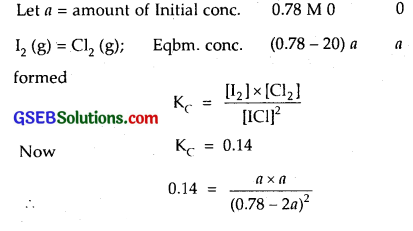On solving for ‘a’
a = 0.21
∴ [I2 (g)] = [Cl2 (g)] = 0.21 M
∴ [ICl] = 0.78 – 0.42 = 0.36 M.Question 17.
Kp = 0.04 atm at 899 K for the equilibrium shown below. What is the equilibrium concentration of C2H6 when it is placed in a flask at 4.0 atm pressure and allowed to come to equilibrium?
C2H6(g) ⇌ C2H4(g) + H2 (g)
Solution: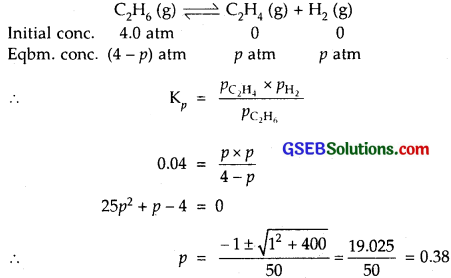∴ Eqbm. cone, of C2H6 (g) = (4.0 – 0.38) atm = 3.62 atm.

Question 18.
Ethyl acetate is formed by the reaction between ethanol and acetic acid and the equilibrium is represented as :
CH3COOH (l) + C2H5OH (l) ⇌ CH3COOC2H5(l) + H2O (l)
(i) Write the concentration ratio (reaction quotient), QC , for this reaction (note : water is not in excess and is not a solvent in this reaction)
(ii) At 293 K, if one starts with 1.00 mol of acetic acid and 0.18 mol of ethanol, there is 0.171 mol of ethyl acetate in the final equilibrium mixture. Calculate the equilibrium constant.
(iii) Starting with 0.5 mol of ethanol and 1.0 mol of acetic acid and maintaining it at 293 K, 0.214 mol of ethyl acetate is found after sometime. Has equilibrium been reached?
Solution:
The given reaction is
CH3COOH (l) + C2H5OH (l) ⇌ CH3COOC2H5(l) + H2O (l)
H2O is not in excess and is hot a solvent.
(i)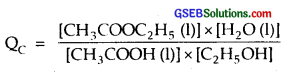(ii) Initial cone.
[CH3COOH(l)] = 1.00 mol
[C2H5OH (l)] = 0.18 mol
[COOC2H5(l)] = 0.171 mol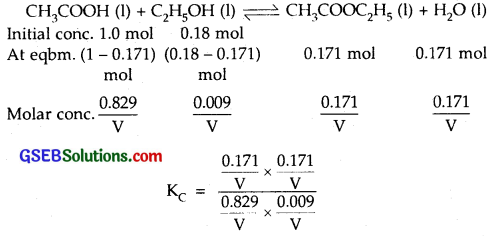where V = vol. of container in L = 3.92
∴ Equilibrium constant = 3.92

(iii)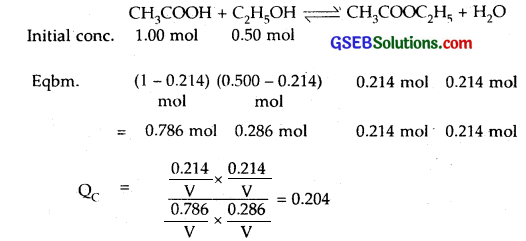As QC ≠ KC
∴ Equilibrium has not been attained.

Question 19.
A sample of pure gCl5 was introduced into an evacuated vessel at 473 K. After equilibrium was attained, concentration of PCl5 was found to be 0.5 x 10-1 mol L-1. If value of KC is 8.3 x 10-3, what are the concentrations of PCl3 and Cl2 at equilibrium?
PCl5 (g) ⇌ PCl3 (g) + Cl2 (g)
Solution:
PCl3 (g) + Cl2 ⇌ PCl5
0.05 mol L-1
Let Eqbm. cone. [PCl3] = [Cl2] = x
As PCl5 dissociates to give PCl3 and Cl2
∴ KC = $$\frac{\left[\mathrm{PCl}_{3}\right] \times\left[\mathrm{Cl}_{2}\right]}{\left[\mathrm{PCl}_{5}\right]}$$
= $$\frac{x \times x}{0.5 \times 10^{-1}}$$
8.3 x 10-3 x 0.5 x 10-1 = x²
or x² = 4 x 10-4 or x = 2 x 10-2 mol K-1
Hence the cone, of PCl3 and Cl2 at eqbm. = 0.02 mol L-1.Question 20.
One of the reaction that takes place in producing steel from iron ore is the reduction of iron (II) oxide by carbon monoxide to give iron metal and CO2.
FeO (s) + CO (g) ⇌ Fe (s) + CO2 (g); Kp = 0.265 atm at 1050 K.
What are the equilibrium partial pressures of CO and CO2 at 1050 K if the initial partial pressures are : pCO = 1.4 atm and PCO2 = 0.80 atm?
Solution: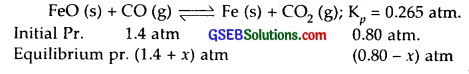Kp = $$\frac{p_{\mathrm{CO}_{2}}}{p_{\mathrm{CO}}}$$ as FeO (s) are solids and their pressures are taken = 1
0.265 = $$\frac { 0.8-x }{ 1.4+x }$$
On solving for x
x = 0.339
∴ Equilibrium partial pr. of CO (PCO) = 1.4 + 0.339 = 1.739 atm.
Equilibrium partial of CO2 [PCO] = 0.800 – 0.339 = 0.461 atm.

Question 21.
Equilibrium constant, KC for the reaction
N2 (g) + 3H2 (g) ⇌ 2NH3 (g)
at 500 K is 0.061 At a particular time, the analysis shows that composition of the reaction mixture is 3.0 mol L-1 N2, 2.0 mol L-1 H2 and 0.5 mol L-1 NH3. Is the reaction at equilibrium? If not in which direction does the reaction tend to proceed to reach equilibrium?
Solution:
The given reaction is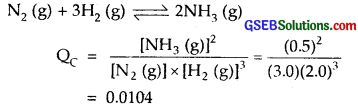Since KC for the reaction is 0.061
As QC < KC
No. the reaction is not at equilibrium.
The reaction will proceed to form more of the products.

Question 22.
Bromine monochloride, BrCl decomposes into bromine and chlorine and reaches the equilibrium :
2BrCl (g) ⇌ Br2 (g) + Cl2 (g)
for which KC = 32 at 500 K. If initially pure BrCl is present at a concentration of 3.3 x 10-3 mol L-1, what is its molar concentration in the mixture at equilibrium?
Solution:
KC = 32 for the reaction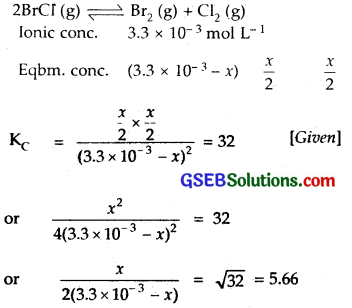Solving for x; x = 3.0 x 10-3
At eqbm. [BrCl] = 3.3 x 10-3 – 3.0 x 10-3 x 10-4 mol L-1
= 3 x 10-4 mol L-1.

Question 23.
At 1127 K and 1 atm pressure, a gaseous mixture of CO and CO2 in equilibrium with solid carbon has 90.55% CO by mass
C (s) + CO2 (g) ⇌ 2CO (g)
Calculate KC for this reaction at the above temperature.
Solution:
The reaction is
C(s) + CO2(g) ⇌ 2C0(g)
If total mass of the mixture of CO and CO2 is 100 g, then
CO = 90.55 g and CO2 = 100 – 90.55 = 9.45 g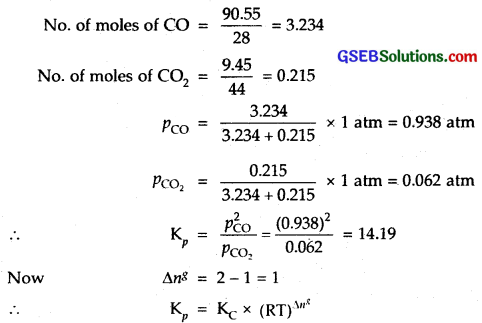where R = 0.0821 L atm K-1 mol-1 T = 1127 K (given)
∆ng = change in the no. of moles of the products – no. of moles of reactants
KC = $$\frac{K_{p}}{R T}$$
= $$\frac { 14.19 }{0.0821 × 1127 }$$
= 0.153

Question 24.
Calculate (a) ∆G° and (b) the equilibrium constant for the formation of NO2 from NO and O2 at 298 K
NO (g) + 1/2 % O2 (g) ⇌ NO2 (g)
where ∆fG° [NO2] = 52.0 kJ/mol
fG° [NO] = 87.0 kJ/mol
fG° [O2] = 0 kJ/mol.
Solution:
(a) The reaction is NO (g) + 1/2 % O2 (g) ⇌ NO2 (g)
∆G° reaction = ∆fG° (NO2) – ∆fG° (NO) – $$\frac {1}{2 }$$∆G°f (O2)
= (52.0 – 87.0) kJ/mol-1
= – 35.0 kJ mol-1.

(b) To calculate KC
– ∆G° = 2.303 RT log KC
– (- 35.0) = 2.303 x 0.08314 x 298 log KC
∴ l0g kC = $$\frac { 35 }{ 5.7058 }$$ = 6.134
∴ KC = antilog 6.134 = 1.361 x 106.Question 25.
Does the number of moles of reaction products increase, decrease or remain same when each of the following equilibria is subjected to a decrease in pressure by increasing the volume?
(a) PC15 (g) ⇌ PCl3 (g) + Cl2 (g)
(b) CaO (s) + CO2 (g) ⇌ CaCO3 (s)
(c) 3Fe (s) + 4H2O (g) ⇌ Fe3O4 (s) + 4H2 (g)
Solution:
(a) PC15 (g) ⇌ PCl3 (g) + Cl2 (g)
Since this reaction proceeds with an increase in volume, therefore when pressure is decreased, the equilibrium will shift in the forward direction and hence more of PCl3 (g) and Cl2 (g) will be formed.

(b) CaO (s) + CO2 (g) ⇌ CaCO3 (s)
This reaction proceeds with a decrease in volume when CaO (s). absorbs CO2 (g) to form CaCO3 (s). Hence when the pressure is lowered, the equilibrium will shift backwards.
Here the no. of moles of the products will decrease.

(c) 3Fe (s) + 4H2O (g) ⇌ Fe3O4 (s) + 4H2 (g)
The presence of Fe (s) and Fe3O4 (s) on the reactant and product side is not influenced by the decrease in pressure. As volume of H2O (g) and H2 (g) are present in the same ratio [4 : 4], there is no effect of decrease of pressure on the number of moles of products, i.e., they remain the same.

Question 26.
Which of the following reactions will get affected by increasing the pressure? Also, mention whether change will cause the reaction to go into forward or backward direction.
(i) COCl2 (g) ⇌ CO (g) + Cl2 (g)
(ii) CH4 (g) + 2S2 (g) ⇌ CS2 (g) + 2H2S (g)
(iii) CO2 (g) + C (g) ⇌ 2CO (g)
(iv) 2H2 (g) + CO (g) ⇌ CH3OH (g)
(v) CaCO3 (s) ⇌ CaO (s) + CO2 (g)
(vi) 4NH3 (g) + 5O2 (g) ⇌ 4NO (g) + 6H2O (g).
Solution:
In all the above reactions, the reaction no. (ii) proceeds with the same no. of moles on both sides
i.e., np = nr = 3
∴ This reaction will not be effected by the increase in pressure
i. e., the direction of equilibrium will not be effected by the increase in pressure.
All other reactions will be effected by the increase in pressure.
(i) COCl2 (g) ⇌ CO (g) + Cl2 (g)
np > nr
np = 2
nr = 1
∴ Equilibrium will shift to the left in increasing pressure.
No effect of increase of pressure.

(iii) CO2 (g) + C (g) ⇌ 2CO (g)
Here nr = 1
np = 2
therefore np > nr
∴Equilibrium will go to left on increase of pressure.

(iv) 2H2 (g) + CO (g) ⇌ CH3OH (g) .
Here nr = 3
np = 1
therefore np < nr
∴ Equilibrium will shift to the right on increasing pressure.

(v) CaCO3 (s) ⇌ CaO (s) + CO2 (g)
Here nr = 0
np = 1
therefore np > nr
∴ Equilibrium will shift backwards (left) on increasing the pressure.

(vi) 4NH3 (g) + 5O2 (g) ⇌ 4NO (g) + 6H2O (g).
Here nr = 9
np = 10
therefore np > nr
∴ Equilibrium will shift backwards on increasing the pressure.Question 27.
The equilibrium constant for the following reaction is 1.6 x 105 at 1024 K
H2 (g) + Br2 (g) ⇌ 2HBr (g)
Find the equilibrium pressure of all gases if 10.0 bar of HBr is introduced into a sealed container at 1024 K.
Solution:
2HBr (g) ⇌ H2 (g) + Br2 (g)
K = $$\frac{1}{1.6 \times 10^{5}}$$
2HBr (g) ⇌ H2 (g) + Br2 (g)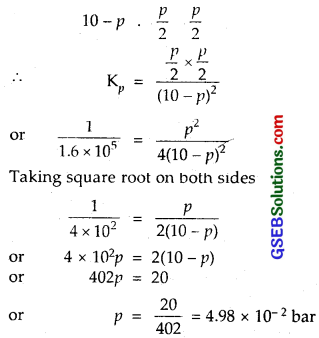Hence at equilibrium $$p_{\mathrm{H}_{2}}$$ = $$p_{\mathrm{Br}_{2}}$$ = $$\frac { p }{ 2 }$$
= 2.5 x 10-2 bar
PHBr = 10 – p = 10 bar.

Question 28.
Dihydrogen gas is obtained from natural gas by partial oxidation with steam as per following endothermic reaction :
CH4 (g) + H2O (g) ⇌ CO (g) + 3H2 (g)
(a) Write an expression of Kp for the above reaction.
(b) How will the values of Kp and composition of equilibrium mixture be affected by
(i) increasing the pressure
(ii) increasing the temperature
(iii) using a catalyst?
Solution:
The given reaction is endothermic (heat is absorbed) and is,
CH4 (g) + H2O (g) ⇌CO (g) + 3H2 (g)
It is proceeding with ah increase in volume (reactants = 2 moles; products = 4 mols)

(a) Kp = $$\frac{p_{\mathrm{CO}} \times p_{\mathrm{H}_{2}}^{3}}{p_{\mathrm{CH}_{4}} \times p_{\mathrm{H}_{2} \mathrm{O}}}$$

(b) (i) By Le Chatelier’s principle will shift in the backward direction on increasing the pressure.

(ii) By Le Chatelier’s principle, it will shift in the forward direction as the reaction is endothermic and is favoured by increase of temperature.

(iii) Equilibrium composition will not be disturbed but equilibrium will be attained quickly.

Question 29.
Describe the effect of :
(c) removal of CO
(d) removal of CH3 OH
on the equilibrium of the reaction :
2H2 (g) + CO (g) ⇌ CH3OH (g)
Solution:
The given reaction is –
2H2 (g) + CO (g) ⇌ CH3OH (g)
(a) Addition of H2 will shift the equilibrium in the forward direction, i.e., more of CH3OH will be formed.
(b) Addition of CH3 OH will shift the reaction backwards, i.e., more of H2 (g) and CO (g) will be formed.
(c) Removal of CO will shift the equilibrium backwards.
(d) Removal of CH3OH will shift the equilibrium forwards.Question 30.
At 473 K, equilibrium constant KC for decomposition of phosphorus pentachloride, PCl5 is 8.3 x 10-3. If decomposition is depicted as
PCl5 (g) ⇌ PCl3 (g) + Cl2 (g); ∆rH° = 124.0 kJ mol-1
(a) write an expression for KC for the reaction
(b) what is the value of KC for the reverse reaction at the same temperature?
(c) what would be the effect on KC if (i) more PCl5 is added (ii) pressure is increased (iii) the temperature is increased?
Solution:
PCl5 (g) ⇌ PCl3 (g) + Cl2 (g); ∆rH° = 124.0 kJ mol-1
KC for the reaction at 473 K = 8.3 x 10-3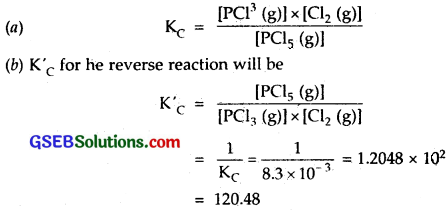(c) (i) If more of PCl5 is added, it will not effect KC as KC is constant at constant temperature.

(ii) No effect.

(iii) As the reaction is endothermic, on increasing the temperature, Kf will increase. As KC = Kf/Kb, it will increase with increase in temperature.

Question 31.
Dihydrogen gas used in Haber’s process is produced by reacting methane from natural gas with high temperature steam. The first stage of two stage reaction involves the formation of CO and Hr In second stage, CO formed in first stage is reacted with more steam in water gas shift reaction.
CO (g) + H2O (g) ⇌ CO2 (g) + H2 (g)
If a reaction vessel at 400°C is charged with an equimolar mixture of CO and steam such that pCO = PH2O = 4.0 bar, what will be the partial pressure of H2 at equilibrium? Kp = 10.1 at 400° C.
Solution:
The given reaction is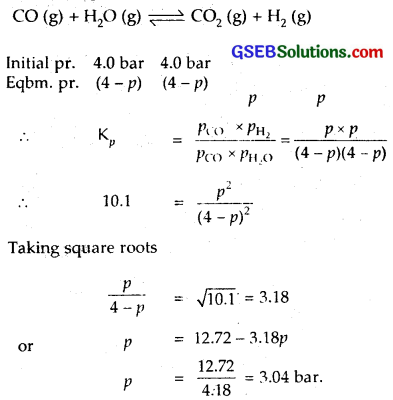Question 32.
Predict which of the following reaction will have appreciable concentration of reactants and. products :
(a) Cl2 (g) ⇌ 2Cl (g); KC = 5 x 10-30
(b) Cl2 (g) + 2NO (g) ⇌ 2NOCl (g); KC = 3.7 x 108
(c) Cl2 (g) + 2NO2 (g) ⇌ 2NO2Cl (g); KC = 1.8
Solution:
(a) As KC = 5 x 10-39. It is very very low
Conc. of Cl2 is high.
∴ cone, of products, i.e., 2 Cl (g) is extremely low.

(b) KC = 3.7 x 108. As value of KC is very high. Therefore the concentration of products, i.e., NOCl is very high.

(c) KC = 1.8; cone, of products, i.e., NO2Cl is somewhat higher than the reactants.Question 33.
The value of KC for the reaction 3O2 (g) ⇌ 2O3 (g) is 2.0 x 10-50 at 25°C. If the equilibrium concentration of O2 in air at 25°C is 1.6 x 10-2, what is the concentration of O3?
Solution:
KC for the reaction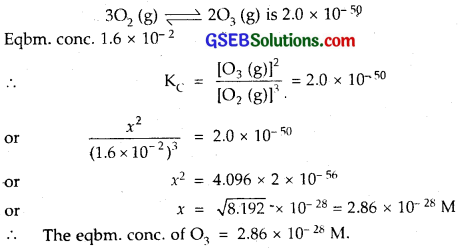Question 34.
The reaction, CO (g) + 3H2 (g) ⇌ CH4 (g) + H2O (g) is at equilibrium at 1300 K in a 1 L flask. It also contain 0.30 mol of CO, 0.10 mol of H2 and 0.2 mol of H2O and an unknown amount of CH4 in the flask. Determine the concentration of CH4 in the mixture. The equilibrium constant, KC for the reaction at the given temperature is 3.90.
Solution:
The given reaction is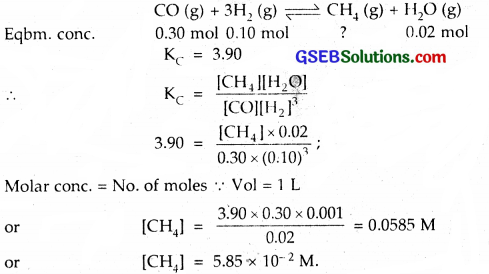Question 35.
What is meant by the conjugate acid-base pair? Find the conjugate acid/base for the following species:
HNO2, CN, HClO4, F, OH, CO32-, and S2-.
Solution:
Conjugate acid-base pair: A conjugate pair of acid and a base differs by a proton only, i.e.,
Conjugate acid ⇌ Conjugate base + H+
HNO2 has a conjugate base NO2
CN has a conjugate acid HCN
HClO4 has a conjugate base ClO4
F has a conjugate acid HF
OH is the conjugate base of H2O. OH has a conjugate base O-2.
CO2-3 is the conjugate base of HCO3 or HCO3 is the conjugate acid of CO32-.
S2- has the conjugate acid HS.

Question 36.
Which of the following are Lewis acids? H2O, BF3 H+, and NH4+.
Solution:
BF3 H+, and NH4+.
[Lewis acid is a substance (molecule or ion) which has a tendency to accept a pair of electrons].

Question 37.
What will be the conjugate bases for the Bronsted acids : HF, H2SO4 and HCO3?
Solution:
HF : its conjugate base is F.
H2SO4 : its conjugate base is HSO4
HCO3 : its conjugate base is CO2-3.

Question 38.
Write the conjugate acids for the following Bronsted bases : NH2, NH3 and HCOO.
Solution:
NH2 its conjugate acid is NH3
NH3 : its conjugate acid is NH+4
HCOO : its conjugate acid is HCOOH.Question 39.
The species : H2O, HCO3, HSO4 and NH3 can act
both as Bronsted acids and bases. For each case give the corresponding conjugate acid and base.
Solution:
(i) H2O has a conjugate acid of H3O+. H2O has a conjugate base of OH.

(ii) HCO3 has a conjugate acid of’H2CO3 and HCO3 has a conjugate base of CO2-3.

(iii) HSO4 has a conjugate acid of H2SO4. HSO4 has a conjugate base of SO2-4.

(iv) NH3 has a conjugate acid of NH+4 . NH3 has a conjugate base of NH2.

Question 40.
Classify the following species into Lewis acids ad Lewis bases and show how these act as Lewis acid/base :
(a) OH
(b) F
(c) H+
(d) BCl3.
Solution:
(a) OH is the Lewis base because it can donate a pair of electrons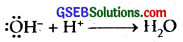(b) $$\overline{F}$$ is the Lewis base as it can furnish a pair of electrons.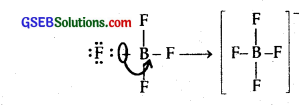(c) H+ is the Lewis acid as it can accept a pair of electrons.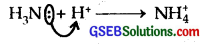(d) BCl3 is the Lewis acid as it can accept a pair of electrons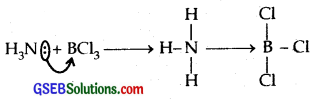Question 41.
The concentration of hydrogen ion in a sample of soft drink is 3.8 x 10-3M. What is its pH?
Solution:
pH = – log [H30+] = – log 3 8 x 10-3
= – (- 3) log 10 – log 3.8
= 3.0 – 0.5798 [log 10 = 1]
pH = 2.42.

Question 42.
The pH of a sample of vinegar is 3.76. Calculate the concentration of hydrogen ion in it.
Solution:
pH = – log [H+]
3.76 = – log [H+]
or log [H+] = – 3.76
= – 3 – 1 + 1 – 0.76 = $$\bar { 4 }$$.24
∴ [H+] = antilog $$\bar { 4 }$$.24
= 1.74 x 10-4 M
Thus hydrogen ion concentration [H+] = 1.74 x 10-4 M.

Question 43.
The ionization constant of HF, HCOOH and HCN at 298 K are 6.8 x 10-4, 1.8 x 10-4 and 4.8 x 10-9 respectively. Calculate the ionization constants of the corresponding conjugate base.
Solution:
Ka x Kb = Ku
where Ka = dissociation ionisation constant of a weak acid
Kb = Ionisation constant of its conjugate base
Ku = Ionic product of water = 1.0 x 10-14 at 298 K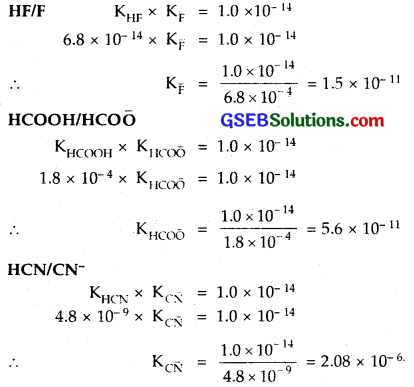Question 44.
The ionization constant of phenol is 1.0 x 10-10. What is the concentration of phenolate ion in 0.05 M solution of phenol? What will be its degree of ionization if the solution is also 0.01 M in sodium phenolate?
Solution:
Ionisation constant of phenol = 1.0 x 10-10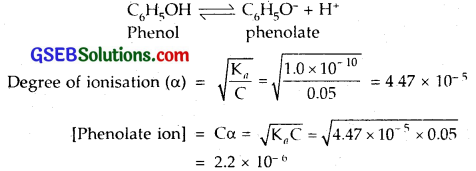In the presence of 0.01 M sodium benzoate, let y be the amount of phenol dissociated, then at eqbm.
[C6H5OH] = 0.05 – y = 0.05;
[C6H5O] = 0.01 + y = 0.01; [H+] = y Mol.
∴ Ka= 0.01 x y/0.05 = 1.0 x 10-10 [Given]
∴ y = 5 x 10-10; α = $$\frac { y }{ c }$$ = 10-8.Question 45.
The first ionization constant of H2S is 9.1 x 10-8. Calculate the concentration of HS ion in its 0.1 M solution. How will this concentration be affected if the solution is 0.1 M in HCl also? If the second dissociation constant of H2S is 1.2 x 10-13, calculate the concentration of S2- under both conditions.
Solution: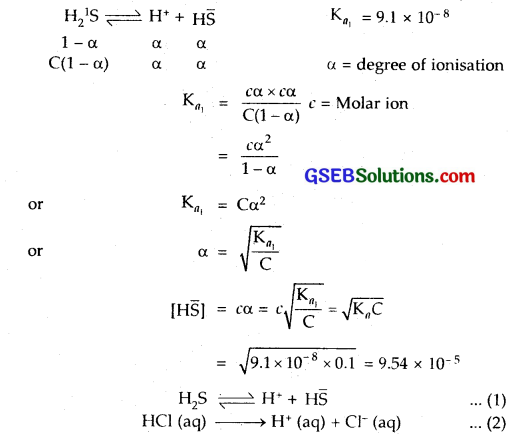In presence of 0.1 M HCl, suppose H2S dissociated is y. Then at eqbm.
[H2S] = (0.1 – y) = y; [H+] = 0.1 + y = 0.1; [ [H$$\bar { S }$$] = y Mol
∴ 9.1 x 10-8 = $$\frac{0.1 \times[\mathrm{HS}]}{0.1}$$ = $$\frac {0.1×y }{ 0.1 }$$
or y = 9.1 x 10-8 M
or [H$$\bar { S }$$] in 0.1 M HCl = 9.1 x 10-8
H2 ⇌ H+ + HS … $$\mathrm{K}_{a_{1}}$$ = 9.1 x 10-8
[H$$\bar { S }$$] ⇌ H+ + S2- $$\mathrm{K}_{a_{2}}$$ = 1.2 x 10-13
∴ Ka = $$\mathrm{K}_{a_{1}}$$ x $$\mathrm{K}_{a_{2}}$$
= 9.1 x 10-8 x 1.2 x 10-13
= 1.092 x 10-20
For the 2nd Case
To calculate [S2-]
Ka = [H+]²[S2-]/[H2S]
In the absence of 0.1 M HCl
[H+] = 2[S2-]
Hence [S2-] = x; [H+] = 2x
∴ $$\frac{(2 x)^{2} \times x}{0.1}$$ = 1.092 x 10-20
or x = 6.5 x 10-8 M
In the presence of 0.1 M HCl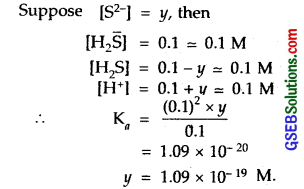Question 46.
The ionization constant of acetic acid is 1.74 x 10-5. Calculate the degree of dissociation of acetic acid in its 0.05 M solution. Calculate the concentration of acetate ion in the solution and its pH.
Solution: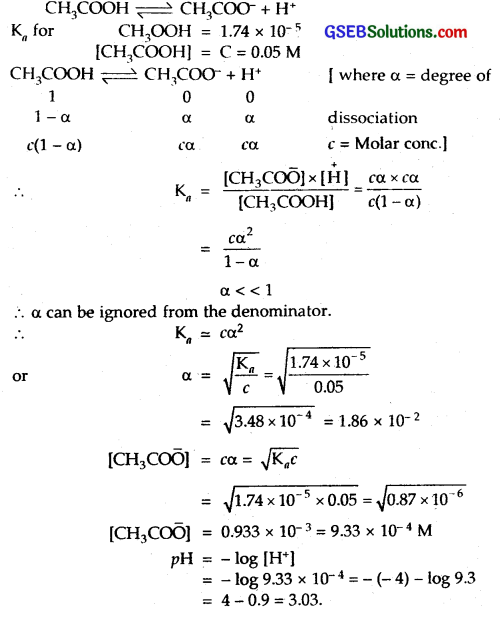Question 47.
It has been found that the pH of a 0.01 M solution of an organic acid is 4.15. Calculate the concentration of the anion, the ionization constant of the acid and its pKa.
Solution:
Organic acid HA ⇌ H+ + A
pH = 4.15 (given); c = [HA] = 0.01 M
∴ [H+] = + 10-pH or – log [H+] = 4.15
log [H+] = – 4.15 = $$\bar { 5 }$$.85
∴ [H+] = antilog $$\bar { 5 }$$.85 = 7.079 x 10-5
∴ Cone, of anion [A] = [H+] = 7.08 x 10-5 M
∴ Ka = (7.08 x 10-5)²/0.01 = 5.08 x 10-7
pKa = – log Ka = – log 5.08 x 10-7= 6.29.

Question 48.
Assuming complete dissociation, calculate the pH of the following solutions :
(a) 0.0O3 M HCl
(b) 0.005 M NaOH
(c) 0.0O2 M HBr
(d) 0.0O2 M KOH.
Solution:
(a) HCl (aq) → Hr+ (aq) + Cl0 (aq)
[HCl] = 0.0O3 M
As HCl is completely dissociated into H+ ions
∴ [H+] = [HCl] = 0.003 M
pH = – log[H+] = – log 3 x 10-3
= -(- 3) log 3 = 3 – log 3 = 3 – 0.477 = 2.523.

(b) NaOH (aq) → Na+ (aq) + OH (aq)
[NaOH] = 0.005 = 5 x 10-3 M
[OH] = [NaOH] = 5 x 10-3 M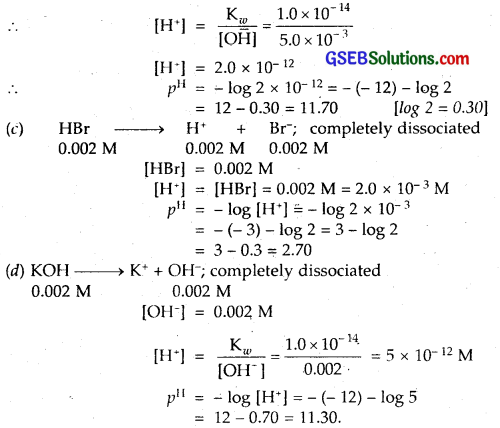Question 49.
Calculate the pH of the following solutions :
(a) 2 g of TlOH dissolved in water to give 2 litre of solution.
(b) 0.3 g of Ca(OH)2 dissolved in water to give 500 mL of solution.
(c) 0.3 g of NaOH dissolved in water to give 200 mL of solution.
(d) 1 ml of 13.6 M HCl is diluted to give 1 litre of solution.
Solution:
(a) TlOH ⇌ Tl+ + OH
[TlOH] = $$\frac { 2 }{ 221×2 }$$ = $$\frac { 1 }{ 221 }$$ m L-1 [TlOH = 204 + 16 + 1 = 221]
∴ [O$$\bar { H }$$] = assuming complete dissociation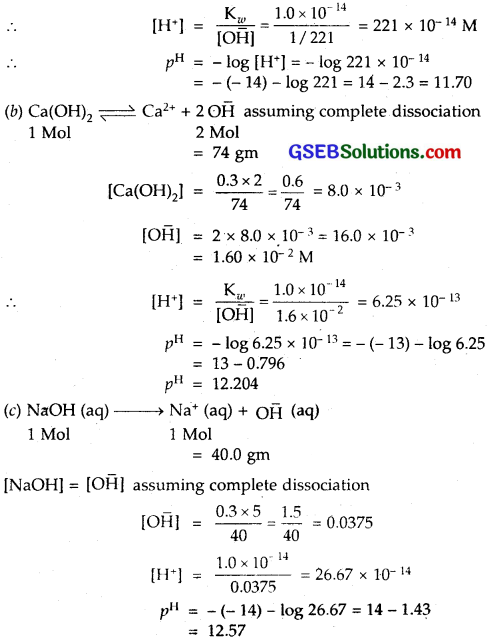(d) 1 ml of 13.6 M HCl is diluted to give 1 litre of solution HCl is completely dissociated to give H+ ions
[HCl] = ?
M1V1 = M2V2
1 ml of 13.6 M HCl = 100 ml of M2 molarity
∴ M2 = $$\frac { 1×13.6 }{ 1000 }$$ = 0.0136 M
∴ [HCl] = [H+] = 0.0136 M
pH = – log [H+] = – log 1.36 x 10-2
= – (- 2) – log 1.36
= 2 – 0.13
= 1.87Question 50.
The degree of ionization of a 0.1 M bromoacetic acid solution is 0.132. Calculate the pH of the solution and the pKa of bromoacetic acid.
Solution:
α (Degree of Ionisation) = 0.132
c (molar cone.) = 0.1 M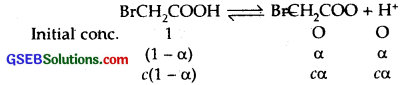∴ [H+] = c x α = 0.1 x 0.132 = 0.0132
pH = – log [H+] = – log 1.32 x 10-2
= – ( – 2) – log 1.32 = 2 – 0.12
= 1.88
pka = – logKa
Now Ka = cα²
Ka = 0.1 x (0.132)² = 1.74 x 10-3
∴ pka = – log 1.74 x 10-3
= – (- 3) – log 1.74
= 3 – 0.24
= 2.76

Question 51.
The pH of 0.005 M codeine [C18H21NO3] solution is 9.95. Calculate its ionization constant and pKb.
Solution:
Molar cone, of codeine = 0.005 M .
pH = 9.95
∴ log [H+] – 9.95 = $$\bar { 10 }$$.05
∴ [H+] = 1.12 x 10-10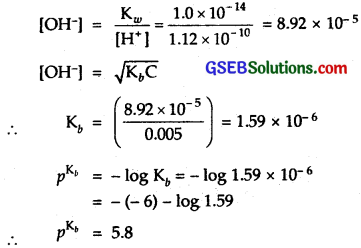Question 52.
What is the pH of 0.001 M aniline solution? The ionization constant of aniline is 4.27 x 10-10. Calculate the degree of ionization of aniline in the solution. Also calculate the ionization constant of the conjugate acid of aniline.
Solution:
Cone, (c) of aniline solution = 0.001 M
Kb (aniline) = 4.27 x 10-10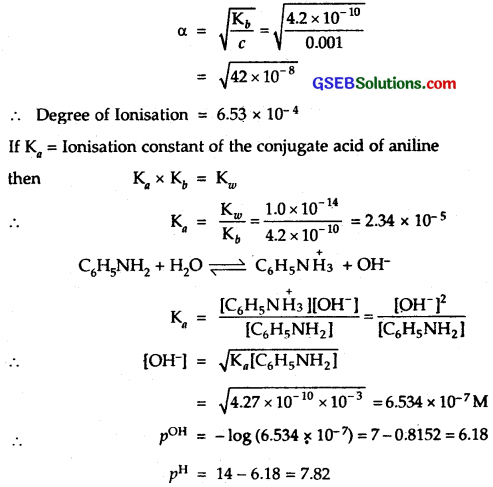Question 53.
Calculate the degree of ionization of 0.05 M acetic acid if its pK value is 4.74. How is the degree of dissociation affected when its solution also contains (a) 0.01 M HCl (b) 0.1 M in HCl?
Solution:
pKa for CH3COOH = 4.74
4.74 = pKa = – log Ka
∴ Ka = antilog $$\bar { 5 }$$.26 = 1.82 x 10-5
Molar cone, of acetic acid = 0.05 M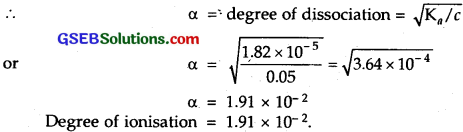(a) To calculate the value of a in presence of 0.01 M HCl
If α is the amount dissociated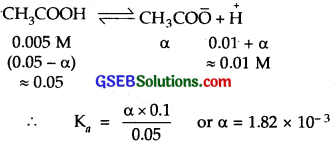(b) In 0.1 M HCl
Let y is the amount dissociated.
[H+] = 0.1 M + y ≈ 0.1 M
α = 1.82 x 10-4Question 54.
The ionization constant of dimethylamine is 5.4 x 10-4. Calculate its degree of ionization in its 0.O2 M solution. What percentage of dimethylamine is ionized if the solution is also 0.1 M in NaOH?
Solution:
Ka for dimethylamine = 5.4 x 10-4
Molar cone. (c) = 0.02 M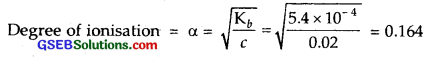(ii) In the presence of 0.1 M NaOH, if x is the amount of dimethylamine dissociated,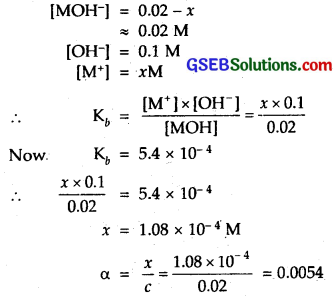Question 55.
Calculate the hydrogen ion concentration in the following biological fluids whose pH are given below :
(a) Human muscle-fluid, 6.83
(b) Human stomach fluid, 1.2
(c) Human blood, 7.38
(d) Human saliva, 6.4.
Solution:
(a) pH of human muscle fluid = 6.83
pH = – log [H+]
6.83 = – log [H+];
[H+] = antilog $$\bar { 7 }$$.17 = 1.479 x 10-7 M

(b) Stomach-fluid
– log [H+] = 1.2
∴ [H+] = antilog 2.8 = 6.3 x 10-2 = 0.063 M.

(c) Human blood
pH = 7.38
– log [H+] = 7.38
or log [H+] = – 7.38 = $$\bar { 8 }$$.62
∴ [H+] = antilog $$\bar { 8 }$$.62 = 4.17 x 10-8 M

(d) Human saliva
pH = 6.4
∴ – log [H+] = 6.4
or [H+ = antilog $$\bar { 7 }$$.6 = 3.98 x 10-7 M]Question 56.
the pH of milk, black coffee, tomato juice, lemon juice and egg white are 6.8, 5.0, 4.2, 2.2 and 7.8 respectively. Calculate corresponding hydrogen ion concentration in each.
Solution:
(a) Milk pH = 6.8
– log [H+] = – 6.8 = $$\bar { 7 }$$.2
∴ [H+] = antilog $$\bar { 7 }$$.2 = 1.5 x 10-7 M.

(b) Black coffee pH = 5.0
– log [H+] = 5.0
log [H+] = – 5 = $$\bar { 5 }$$.0000
∴ [H+] = 10-5 M.

(c) Tomato juice pH = 4.2
– log [H+] = 4.2
∴ log [H+] = – 4.2= $$\bar { 5 }$$.8
∴ [H+] = antilog $$\bar { 5 }$$.8 = 6.31 x 10-5 M.

(d) Lemon juice pH = 2.2
– log [H+] = 2.2
log [H+] = – 2.2 = $$\bar { 3 }$$.8
[H+] = antilog $$\bar { 3 }$$.8 = 6.31 x 10-3 M.

(d) Egg-white pH = 7.8
– log [H+] = 7.8
∴ log [H+] = – 7.8 = $$\bar { 8 }$$.2
[H+] = antilog $$\bar { 8 }$$.2 = 1.5 x 10-8 M.

Question 57.
If 0.561 g of KOH is dissolved in water to give 200 mL of solution at 298 K. Calculate the concentrations of potassium, hydrogen and hydroxyl ions. What is its pH?
Solution:
Molar cone, of KOH = $$\frac{0.561 \times 1000}{56.1 \times 200}$$ = 0.05 M
KOH, being a strong electrolyte, is completely ionised in aqueous solution.
∴ KOH (aq) → K+ (aq) + O$$\bar { H }$$ (aq)
[KOH] = [K+] = [OH] = 0.05 M
Now [H+] = $$\frac{\mathrm{K}_{w}}{\left[\mathrm{OH}^{-}\right]}$$
= $$\frac{1.0 \times 10^{-14}}{0.05}$$
= 2.0 x 10-13 M
∴ pH = – log [H+] = – log 2.0 x 10-13
= – (- 13) – log 2 = 13 – 0.30
pH = 12.7

Question 58.
The solubility of Sr[OH]2 at 298 K is 19.23 g/L of solution. Calculate corresponding hydrogen ion concentration in each.
Solution:
The solubility of Sr (OH)2 at 298 K = 19.23 g L-1
Molar concentration dissolved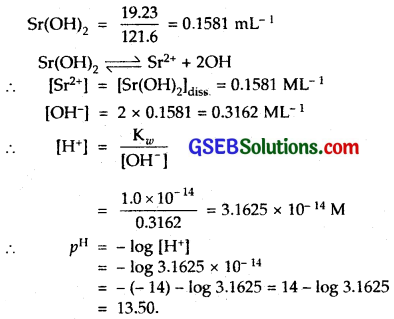Question 59.
The ionization constant of propanoic acid is 1.32 x 10-5. Calculate the degree of ionization of the acid in its 0.05 M solution and also its pH. What will be its degree of ionization if the solution is 0.01 M in HCl also?
Solution: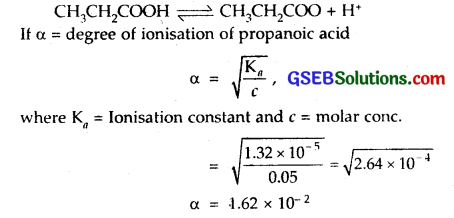∴ degree of ionisation = 1.62 x 10-2
[H+] = cα = 0.05 x 1.62 x 10-2
= 8.1 x 10-4
pH = – log [H+] = – log 8.1 x 10-4
= – (- 4) – log 8.1
= 4 – log 8.1
pH = 3.09.
Degree of ionisation if the solution is 0.01 M in HCl also.
In presence of HCl,. eqbm. will shift in backward direction i.e., concentration of CH3CH2COOH will decrease.
If c is the initial concentration and x is the amount of CH3CH2COOH now dissociated, then at eqbm.
[CH3CH2COOH] = c – x
[CH3CH2CO$$\bar { O }$$] = x; [H+] = 0.01 + x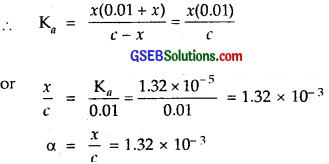Question 60.
The pH of 0.1 M solution of cyanic acid [HCNO] is 2.34. Calculate the ionization constant of the acid and its degree of ionization in the solution.
Solution:
pH = 2.34
Molar cone, (c) = 0.1 M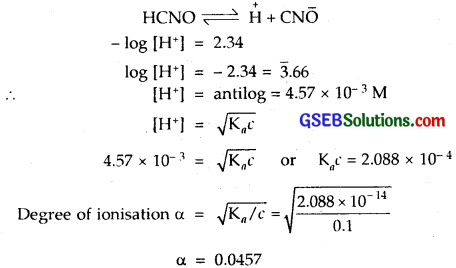Question 61.
The ionization constant of nitrous acid is 4.5 x 10-4. Calculate the pH of 0.04 M sodium nitrite solution and also its degree of hydrolysis.
Solution:
Hydrolysis constant Kh = $$\frac{\mathrm{K}_{w}}{\mathrm{~K}_{a}}$$
where Kw = Ionic product of water, Ka = Ionisation constant of the acid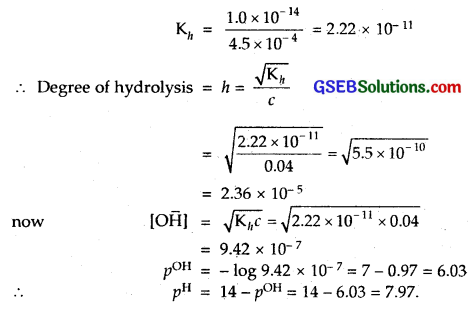Question 62.
A 0.02 M solution of pyridinium hydrochloride has pH = 3.44. Calculate the ionization constant of pyridine.
Solution:
Pyridinium hydrochloride is the salt of a vyeak base pyridine and a strong acid HCl. Hence,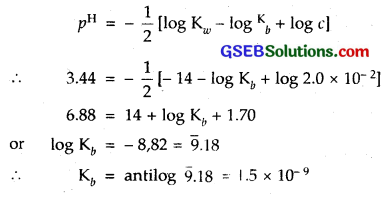Question 63.
Predict if the solutions of the following salts are neutral, acidic or basic :
NaCl, KBr, NaCN, NH4NOJ, NaNO2 and KF.
Solution:

• NaCl : Its solution is neutral as it is the salt of a strong acid and a strong base.
• KBr : Its solution is neutral as it is the salt of strong acid HBr and a strong base KOH.
• NaCN : Its solution is basic as it is the salt of a strong base NaOH and a weak acid HCN.
• NH4NO3 : Its solution is acidic as it is the salt of a strong acid HNO3 and a weak base NH4OH.
• NaNO2 : Its solution is basic as it is the salt of a strong base NaOH and a weak acid HNO2.
• KF : Its solution is basic as it is the salt of a weak acid HF and a strong base KOH.Question 64.
The ionization constant of chloroacetic acid is, 1.35 x 10-3. What will be the pH of 0.1 M acid and its 0.1 M sodium salt solution?
Solution:
The given reaction is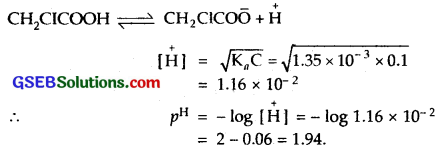Sodium salt of chloroacetic acid is a salt of strong base NaOH and a weak acid chloroacetic acid [CH2ClCOOH]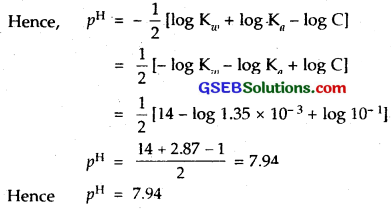Question 65.
Ionic product of water at 310 K is 2.7 x 10-14. What is the pH of neutral water at this temperature?
Solution:
At 310 K; Ionic product of water
Kw = 2.7 x 10-14
∴ [H+ x [OH] = 2.7 x 10-14
But in neutral water [H+] = [OH]
∴ [H+]² = 2.7 x 10-14
or [H+] = $$\sqrt{2.7 \times 10^{-14}}$$ = 1.64 x 10-7 M
PH = – log [H+] = – log 1.64 x 10-7
= – (- 7) – log 1.64
= 7 – 0.215
= 6.785.

Question 66.
Calculate the pH of the resultant mixtures :
(a) 10 mL of 0.2 M Ca[OH]2 + 25 mL of 0.1 M HCl
(b) 10 mL of 0.01 M H2SO4 + 10 mL of 0.01 M Ca[OH]2
(c) 10 mL of 0.1 M H2SO4 + 10 mL of 0.1 M KOH.
Solution:
(a) 10 mL of 0.2 M Ca(OH)2 = 10 x 0.2 millimoles = 2 millimoles
25 mL of 0.1 M HCl – 25 x 0.1 millimoles
= 2.5 millimoles
Ca(OH)2 + 2 HCl → CaCl2 + 2H2O
0.1 millimoles of Ca(OH)2 reacts with 2 millimoles of HCl
∴ 2.5 millimoles of HCl will react with 1.25 millimoles of Ca(OH)2
∴ Ca(OH)22 left – 2 – 1.25 = 0.75 millimoles
Total volume of the solution = 10 + 25 = 35 mL
∴ Molarity of Ca(OH)2 in the mixture solution
= $$\frac { 0.75 }{ 35 }$$ M = 0.0214 M
∴ [OH] = 2 x 0.O214 = 0.O428 M
= 4.28 x 10-2 M
pOH = – log 4.28 x 10-2 = 1.37
∴ pH = 14 – 1.37 = 12.63

(b) 10 mL of 0.01 M H2SO4 will give 10 mL of 0.02 M H+
10 mL of 0.01 M Ca(OH)2 will give 10 ml of 0.02 M OH
10 ml of 0.02 M H+ will neutralize 10 ml of 0.02 M OH ions
There will be left no H+ ions or OH -ions of acid or base
∴ Solution will be neutral on its pH = 7.

(c) 10 ml of 0.1 M H2SO4 will give 10 ml of 0.2 M H+
10 ml of 0.1 M KOH will give 10 ml of 0.1 M O$$\bar { H }$$
∴ Solution will be left with 0.1 M H+ in 10 mL
[H+] = $$\frac { 0.1×10 }{ 1000 }$$ = 1.0 x 10-3
Hence pH = – log [H+] = – log 10-3 = 3

Question 67.
Determine the solubilities of silver chromate, barium* chromate, ferric hydroxide, lead chloride and mercurous iodide at 298 K from their solubility product constants given in Table 7.9. Determine also the molarities of individual ions.
Solution: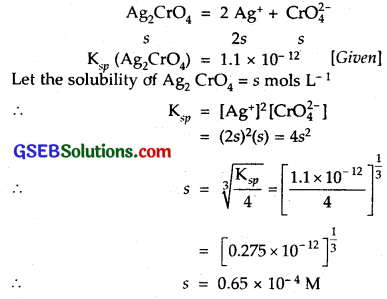Molarity of Ag+ = 2s = 1.30 x 10-4 M and CrO2-4 = 0.65 x 10-4.
Barium Chromate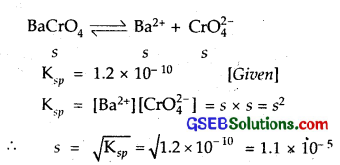Molarity of both Ba2+ and CrO2- 4 = s = 1.1 x 10-5 M
Ferric hydroxide Fe(OH)3 ⇌ Fe3+ + 3OH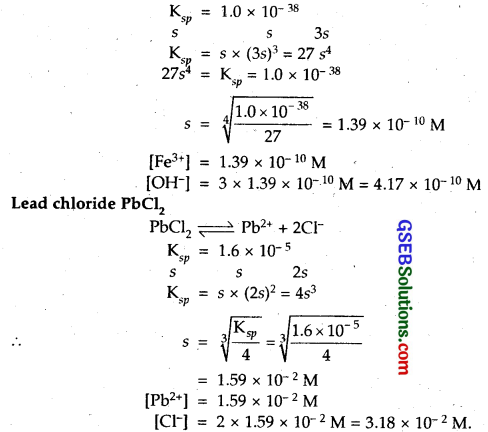Mercurous Iodide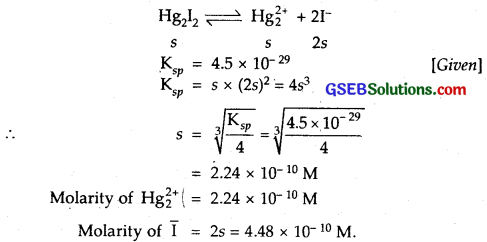Question 68.
The solubility product constant of Ag2CrO4 and AgBr are 1.1 x 10-12 and 5.0 x 10-13 respectively. Calculate the ratio of the molarities of their saturafed solutions.
Solution:
Suppose the solubility of AgBr is s M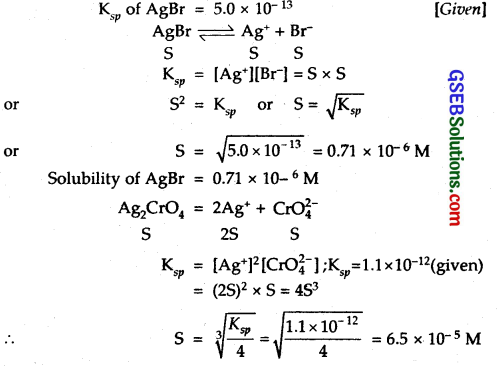∴ Solubility of Ag2CrO4 = 6.5 x 10-5 M
It is clear from above that solubility of Ag2CrO4 is more than that of AgBr.
The ratio of their molarities in their saturated solutions is 91.9 in favour of Ag2CrO4.

Question 69.
Equal volumes of 0.002 M solutions of sodium iodate and cupric chlorate are mixed together. Will it lead to precipitation of copper iodate? (For cupric iodate Ksp = 7.4 x 10-8).
Solution:
2NaIO3 + Cu(ClO3)2 → Cu(IO3)2 + 2NaClO3
Molar cone, of both solutions before mixing = 0.002.
Molar cone, of both solutions after mixing
[IO3] = [Cu2+] = $$\frac { 0.002 }{ 2 }$$ = 0.001 M
(as they have equal volumes)
2[IO3 + Cu2+ → Cu(IO3)2
Ksp of (CuIO3)2 = 7.4 x 10-8
[Cu2+] = 0.001 M
[IO3] = 0.001 M
Ionic Product = [Cu2+] [IO3
= 10-3 x (1 x 10-3)² = 1.0 x 10-9.
Since the Ionic product is less than the solubility product.
∴ No precipitation will occur.Question 70.
The ionization constant of benzoic acid is 6.46 x 10-5 and Ksp for silver benzoate is 2.5 x 10-13. How many times is silver benzoate more soluble in a buffer of pH 3.19 compared to its solubility in pure water?
Solution:
C6H5COO Ag ⇌ C6H5 CO$$\bar { O }$$ + Ag+
Solubility in water
Suppose solubility of silver benzoate in water = x mol -L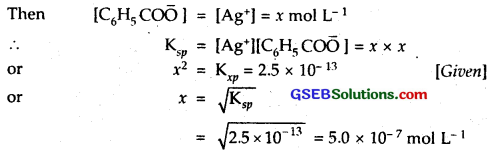Solubility in buffer of pH = 3.19
3.19 = – log [H+]
∴ log [H+] = – 3.19 = $$\bar { 4 }$$.81
∴ [H+] = antilog 4.81
[H+] = 6.457 x 10-4
C6H5 CO$$\bar { O }$$ ions now combine with H+ ions to form benzoic acid but [H+] remains almost constant because we have buffer solution.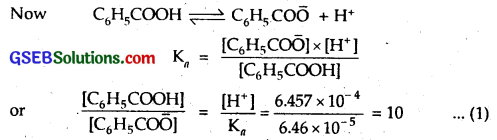Suppose solubility in the buffer solution is y mol L-1. Then as most of the benzoate ions are converted to benzoic acid molecules (which remain almost ionised), we have
V = [Ag+] = [C6H5COO]+[C6H5COOH]
= [C6H5 CO$$\bar { O }$$] + 10[C6H5COOH],
using equation (1) above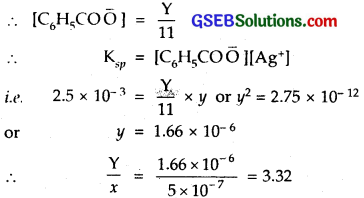Thus silver benzoate is 3.32 times more-soluble at lower pH.

Question 71.
What is the maximum concentration of equimolar solutions of ferrous sulphate and sodium sulphide so that when mixed in equal volumes, there is no precipitation of iron sulphide? (For iron sulphide, K = 6.3 x 10-18).
Solution:
Suppose the concentration of each of FeSO4 and Na2S is x mol L-1. Then after mixing equal volumes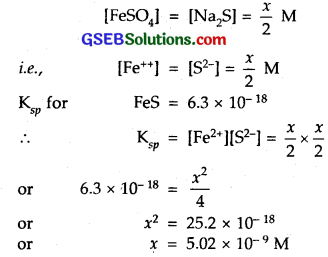Thus the highest molarity for the solution is 2.5 x 10-9 M.Question 72.
What is the maximum volume of water required to dissolve 1 g of calcium sulphate at 298 K? (For calcium sulphate, Ksp is 9.1 x 10-6).
Solution:
CaSO4 (s) ⇌ Ca² (aq) + SO2- (aq)
If s is the solubility of CaSO4 in mol L-1 then
Ksp = [Ca2+][SO2-4]
= s x s = s²
Ksp for CaSO4 = 9.1 x 10-6
∴ s² = 9.1 x 10-6
or s = $$\sqrt{9.1 \times 10^{-6}}$$
= 3.02 x 10-3 mol L-1
Now molecular mass of CaSO4
= 40 + 32 + 4 x 16 = 136 g
∴ s = 3.02 x 10-3 x 136 g L-1 = 0.41 g L-1
Thus for dissolving 0.411 minimum water required = 1 L
∴ For‘dissolving 1 gm, water required = L$$\frac { 1 }{ 0.411 }$$ L = 2.46 L.

Question 73.
The concentration of sulphide ion in 0.1 M HCl solution saturated with hydrogen sulphide is 1.0 x 10-19 M. If 10 mL of this is added to 5 mL of 0.04 solution of the following : FeSO4, MnCL2 ZnCl2 and CdCl2 in which of these solutions precipitation will take place?
Solution:
Precipitation will take place in the solution for which ionic product is greater than the solubility product. As 10 mL of solution containing S2- ion is mixed with 5 mL of metal salt solution, after mixing.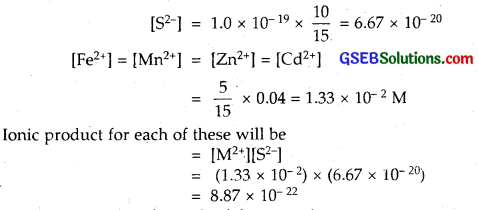Thus Ionic product for each of these species
= 8.87 x 1-22
This value of Ionic product is greater than the solubility products (Ksp) of ZnS (1.6 x 10-24) and CdS (8.0 x 10-27) and less than the values of FeS (6.3 x 10-18) and that of MnS (2.5 x 10-13)
Therefore out of the 4 species, only ZnCl2 and CdCl2 solutions will be precipitated.